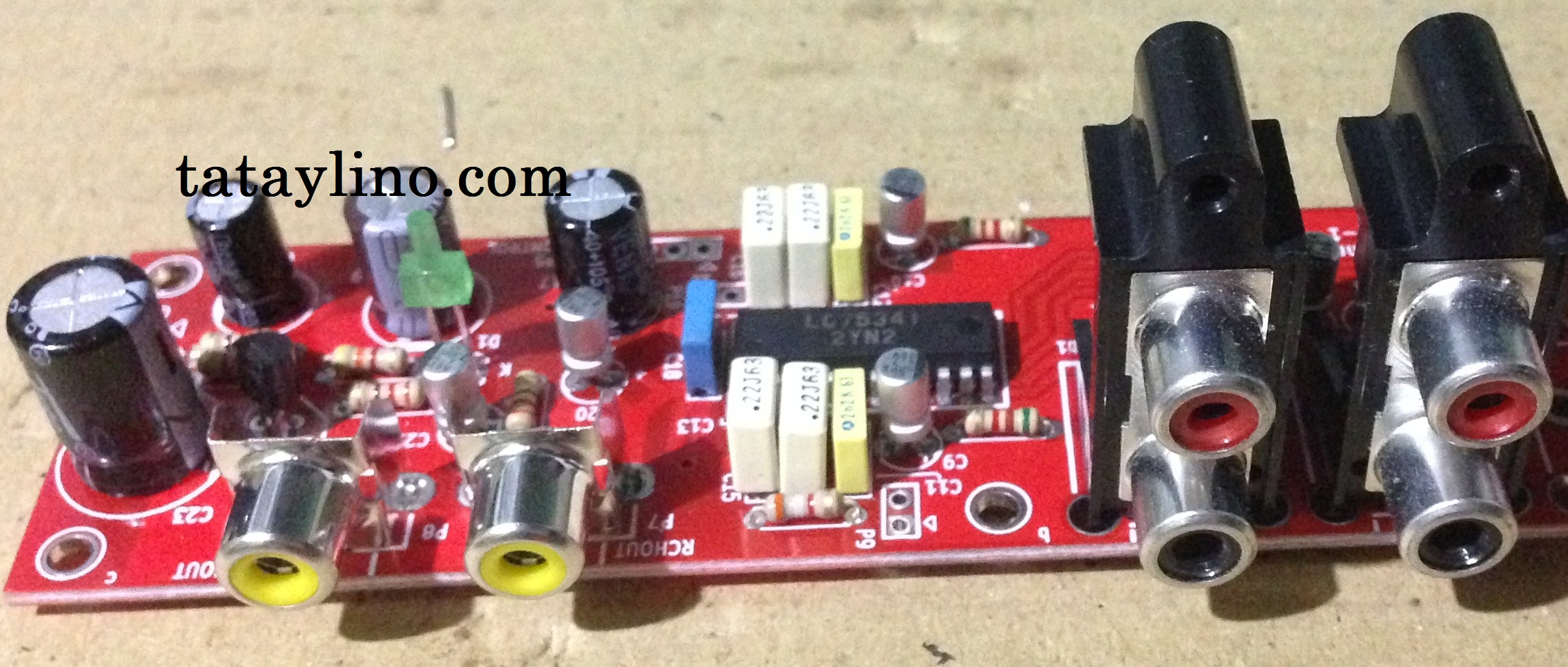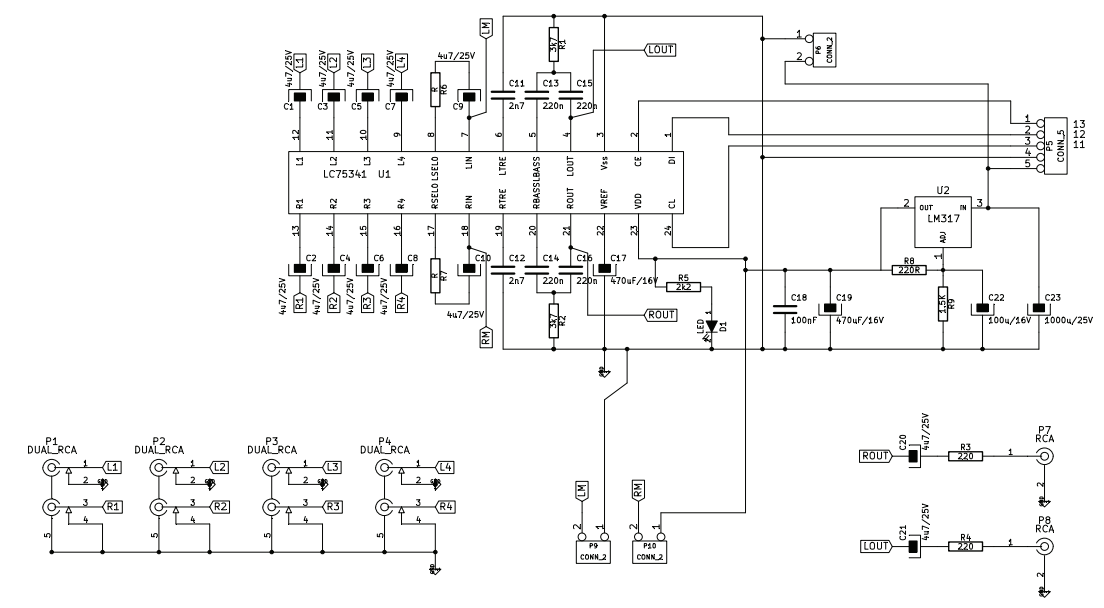# LC75341 tone control using arduinoLC75341 is a digitally controlled analog tone control for audio applications. You can control parameters such as Bass, Treble, Volume and gain through its digital serial data transfer. The data is a 40 bits wide, 8 bits for the address and the 32 bits is the controls data. It is very unusual and you cannot use the microcontrollers built in UART, I2C, etc. I can’t find anywhere on the internet any examples of programs to control this particular IC so I made one for myself. I want to share the code for anyone who want to build this kind of project. The code is simple and I will only share the part of the code that sends data control to the chip. It is up to you to modify it according to your needs.

Microcontroller

The microcontroller use is from ATMEL Atmega328. I am used to PIC microcontrollers, but looking at the arduino nano, it seems a better choice for this project. It has a built-in USB bootloader and has higher memory. Arduino also has more sample programs and tutorials online. It is my first time to use arduino and I am very much surprise how easy to use and program it.

Schematic

For this one I use the digital tone control kit bought from E-Gizmo. I have no time to make my own PCB so I decided to buy a ready made PCB kit. The circuit uses LC75341 tone control IC.Arduino Program

The program is simple, I will only share the control part.

// LC75341 Tone Control program for Arduino by Tataylino.com
const int CE = 2; // CE pin
const int DI = 3; // DI pin
const int CL = 4; // CL pin
const int LED = 13; // CL pin
long dataset;
unsigned char n = 0;

// set variables
unsigned int bass_level = 0;
unsigned int treble_level = 0;
unsigned int input = 1;
unsigned int input_gain = 0;
unsigned int RL = 3;
unsigned int vol = 0;
void setup()
{
pinMode(CE, OUTPUT);
pinMode(DI, OUTPUT);
pinMode(CL, OUTPUT);
pinMode(LED, OUTPUT);
for (n = 0; n < 33; n++)
{
dataset[n] = 0;
}
}

void loop()
{
digitalWrite(LED, HIGH); // This is only to blink the LED

// Set variables
input = 1; // 1 – 4
input_gain = 15; // 0- 15
vol = 80; // 0 – 80 (actual value is negative, 0 = loudest)
treble_level = 13; // 0 – 11 (odd numbers =  negative)
bass_level = 10; // 0 – 10
RL = 3; // 1 – 3

input_select(input);
SetGain(input_gain);
SetVolume(vol);
SetTreble(treble_level);
SetBass(bass_level);
SelectCh(RL);

sendcommand(); // send command to LC75341
delay(300);
digitalWrite(LED, LOW); // This is only to blink the LED
delay(500);
}

void sendcommand()
{
n = 0;
digitalWrite(CE, LOW);
for (n = 0; n < 8; n++)
{
digitalWrite(CL, LOW);
delayMicroseconds(7);
digitalWrite(CL, HIGH);
delayMicroseconds(1);
}
delayMicroseconds(7);
digitalWrite(CL, LOW);
digitalWrite(CE, HIGH);

for (n = 0; n < 32; n++)
{
//send data
digitalWrite(DI, dataset[n]);
digitalWrite(CL, LOW);
delayMicroseconds(7);
digitalWrite(CL, HIGH);
delayMicroseconds(1);
}
delayMicroseconds(12);
digitalWrite(CL, LOW);
digitalWrite(DI, LOW);
delayMicroseconds(7);
digitalWrite(CE, LOW);
}

void input_select(int InputCh)
{
/*
D0-D3 input switch control
0000 – LR1
1000 – LR2
0100 – LR3
1100 – LR4
other combinations = all off
*/
unsigned char x = 0;
if(InputCh == 1)
{
x = 0;
} else if(InputCh == 2)
{
x = 2;
} else if(InputCh == 3)
{
x = 1;
} else //ch = 4
{
x = 3;
}
}

void SetGain(int gain)
{
/*
D4 – D7 input Gain
0000 – 0dB
1000 – +2dB
0100 – +4dB
1100 – +6dB

1111 – +30dB
*/
if(gain > 15) // set saturation limits
{
gain = 15;
}
}

void SetVolume(int level)
{
/*
D8 – D15 Volume Control
00000000 – 0dB
10000000 – -1dB
01000000 – -2dB
11000000 – -3dB
…
11110010 – -79dB
00001010 – infinite
*/
if(level > 80)
{
level = 80;
}
}

void SetTreble(int treble)
{
/*
D16 – D19 Treble Control
1100 – +6dB
0100 – +4dB
1000 – +2dB
0000 – 0dB
1001 – -2dB
0101 – -4dB

1011 – -10dB
*/
}

void SetBass(int bass)
{
/*
D20 – D25 Bass Control
000000 – 0dB
101000 – +2dB
010000 – +4dB
110000 – +6dB
001000 – +8dB

010100 – +20dB
*/
}

void SelectCh(int ch)
{
/*
D26 – D27 Channel selection
00 –
10 – Rch
01 – Lch
11 – Both
*/